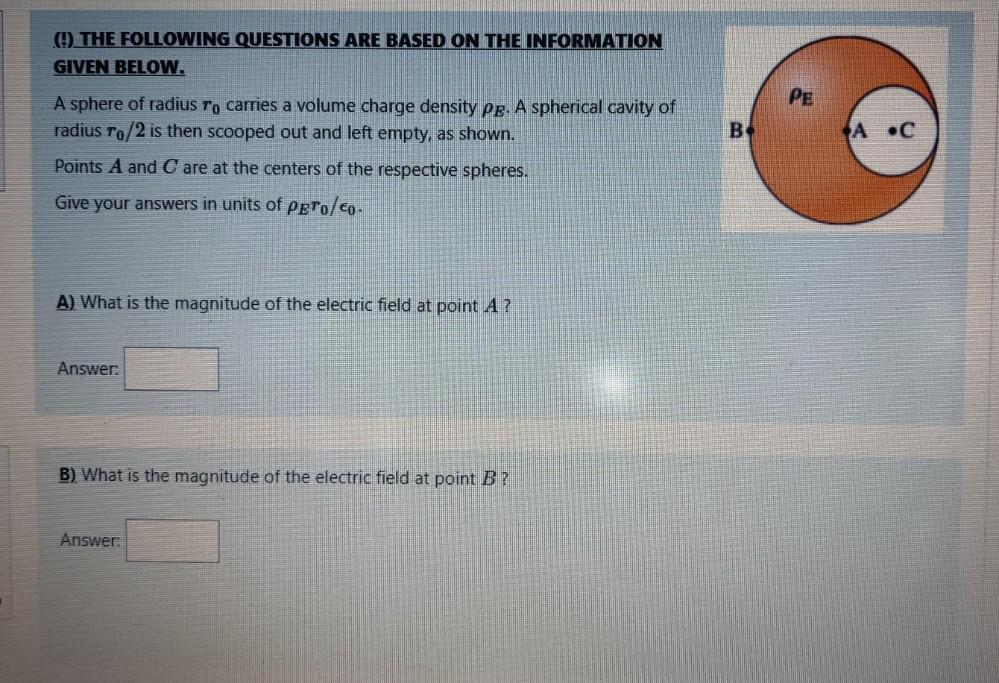Question:

# ( THE FOLLOWING QUESTIONS ARE BASED ON THE INFORMATION GIVEN BELOW. B A •C A sphere of radius To carries a volume charge density( THE FOLLOWING QUESTIONS ARE BASED ON THE INFORMATION GIVEN BELOW. B A •C A sphere of radius To carries a volume charge density pe. A spherical cavity of radius to/2 is then scooped out and left empty, as shown. Points A and C are at the centers of the respective spheres. Give your answers in units of Pero/co. A). What is the magnitude of the electric field at point A ? Answer: B) What is the magnitude of the electric field at point B ? Answer: